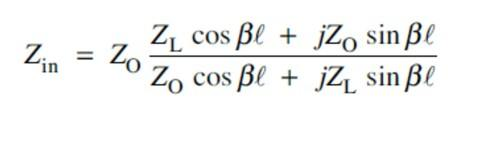# Transmission line input impedance

• acw260

## Homework Statement

(a) A transmission line has a length, ℓ, of 0.4λ. Determine the phase change, βℓ, that occurs down the line.
(b) A 50Ω lossless transmission line of length 0.4λ is terminated in a load of (40 + j30) Ω. Determine, using the equation given below, the input impedance to the line.

[see attachment for equation]

As above.

## The Attempt at a Solution

(a) β = ω / fλ
∴ βℓ = ω 0.4λ / fλ
= ω 0.4 / f
= 0.4x2π f / f
= 0.8π (=2.513 to 3d.p.)

(b) Zin = 50 x [ (40cos0.8π) / ( 50cos0.8π + 30sin0.8π) ]
= 70.91 ohms

Do these answers look correct? Seems worryingly straightforward.

#### Attachments

• Input impedance formula.pdf
46.5 KB · Views: 587

I think you have to consider the reflection from the mismatched termination.

Ok, I can see that I've manipulated the given formula incorrectly for (b). Second attempt:

Zin = Zo [ ( (ZL COS βℓ) + (jZo SIN βℓ) ) / ( (Zo COS βℓ) + (jZL SIN βℓ) ) ]

= 50 x [ ( (40+j30)COS(0.8π) + (j50)SIN(0.8π) ) / ( 50COS(0.8π) + j(40+j30)SIN(0.8π) ) ]

= 25.47 + j5.9

How does this look?

## Homework Statement

(a) A transmission line has a length, ℓ, of 0.4λ. Determine the phase change, βℓ, that occurs down the line.
(b) A 50Ω lossless transmission line of length 0.4λ is terminated in a load of (40 + j30) Ω. Determine, using the equation given below, the input impedance to the line.

[see attachment for equation]

As above.

## The Attempt at a Solution

(a) β = ω / fλ
∴ βℓ = ω 0.4λ / fλ
= ω 0.4 / f
= 0.4x2π f / f
= 0.8π (=2.513 to 3d.p.)
OK. What was with the ω and f? β = 2π/λ so βl = (2π/λ)(0.4λ) = 2.513 radians.
b) I didn't check your math but the formula you used in your later post looks right.

Hello there, I am struggling with the maths of the equation for b.) Zin = Zo [ ( (ZL COS βℓ) + (jZo SIN βℓ) ) / ( (Zo COS βℓ) + (jZL SIN βℓ) ) ] I have attached my working out and have computed the answer with my calculator in Radians but am not at all confident on the answer I have achieved.

#### Attachments

• Equation 1.pdf
874.2 KB · Views: 832
You should have reduced numerator and denominator without changing to polar coordinates:

Z = {(a+jb) + (c+jd)} / {e+jf) + (g+jh)} = {(a+c) + j(b+d)} / {(e+g) + j(f+h)}

THEN changed numerator and denominator to polar.
Rule: for add & subtr, work in rectangular; for mult. & divide, work in polar.
I won't check your math but I suggest you redo using the above stratagem.

hi wanted to put this on as a new post but not sure how so thought it best to place with relevant question

(b) A 50Ω lossless transmission line of length 0.4λ is terminated in a load of (40 + j30)Ω. determine, using the equation given below, the input impedance to the line.I have calculated Bl which I think is correct and converted the load Zl to polar.

βl + 2π x 0.4 = 0.8π
βl = 2.513 radians = 144°

ZL = 40 + j30Ω
ZL = 50∠36.87°

Am I correct in thinking that the 50Ω of the lossless transmission line is used as the Zo.

once put into the formula the result I get is Zin = 50∠36.87, 40 + j30Ω which is equal to the load which makes me think it is incorrect.

thanks

Am I correct in thinking that the 50Ω of the lossless transmission line is used as the Zo.
yes
once put into the formula the result I get is Zin = 50∠36.87, 40 + j30Ω which is equal to the load which makes me think it is incorrect.
thanks
i can't check your math but you're on the right track

Thank you rude man for the very fast response, I keep doubting the answers I'm getting and keep trying to find ways to double check my results. I will continue with the assignment and hopefully get it completed soon. cheers

There is a point in the equation where j29.5/_36.87 needs to be changed to rectangular form.

This would be J29.5cos(36.87)=j23.6 and J29.5sin(36.87)=JJ17.7

It's at this point JJ17.7 is equal to minus 17.7 and it throws the answer that you are getting out?

If JJ17.7 was equal to +17.7 I get to your answer but j squared x 17.7 is a minus and from there I am not getting to an answer where ZL is equal in Zin.

Am I missing something? :-(

Hi Matt, I only handed the assignment In last Saturday so have just checked if I have yet received any response and I have. it has been highlighted that I have made a sign error and it should have been a minus so you are probably correct. I have just recalculated my equation and the result I now have is 26.143∠13.05, 25.466 + j5.912. is that anything like your answer. cheers

@casper12, instead of "hijacking" a thread that is almost two years old, for future questions, please start a new thread. At the top level of this and other five homework forum sections, there's a button labeled "Post New Thread." Clicking that button presents you with the homework template, which you use to enter the problem statement, relevant formulas, and your work. Do not delete any part of the homework template.